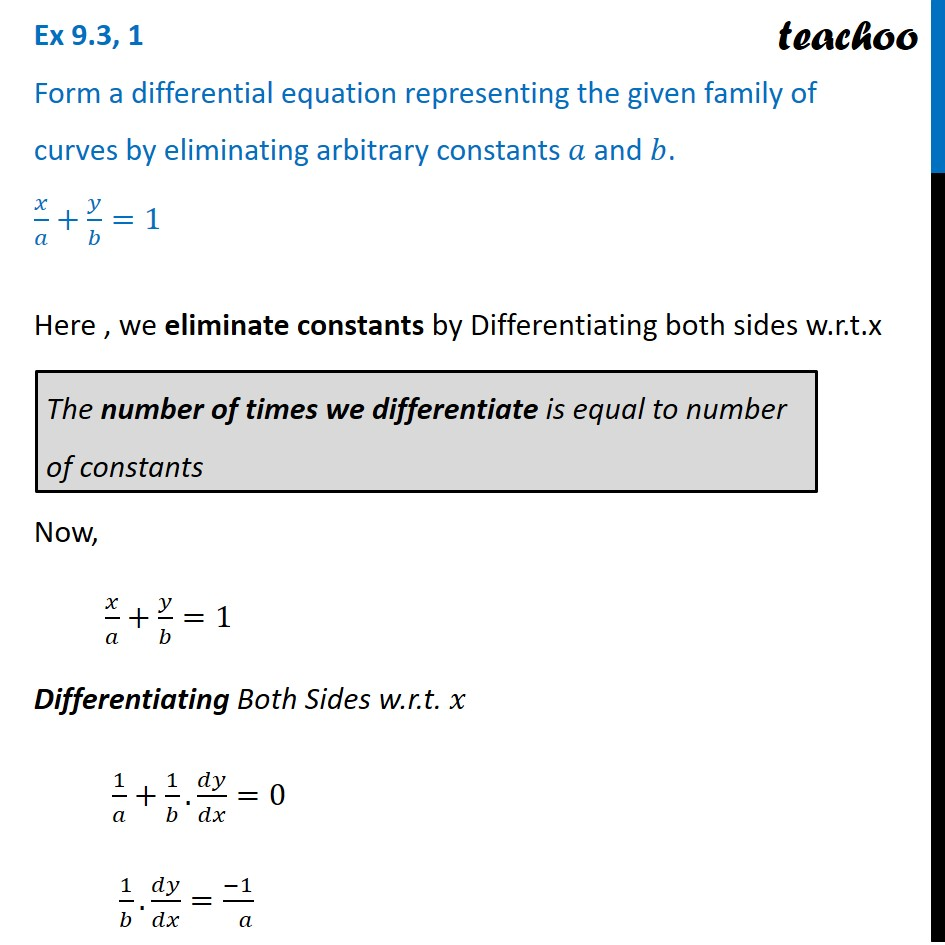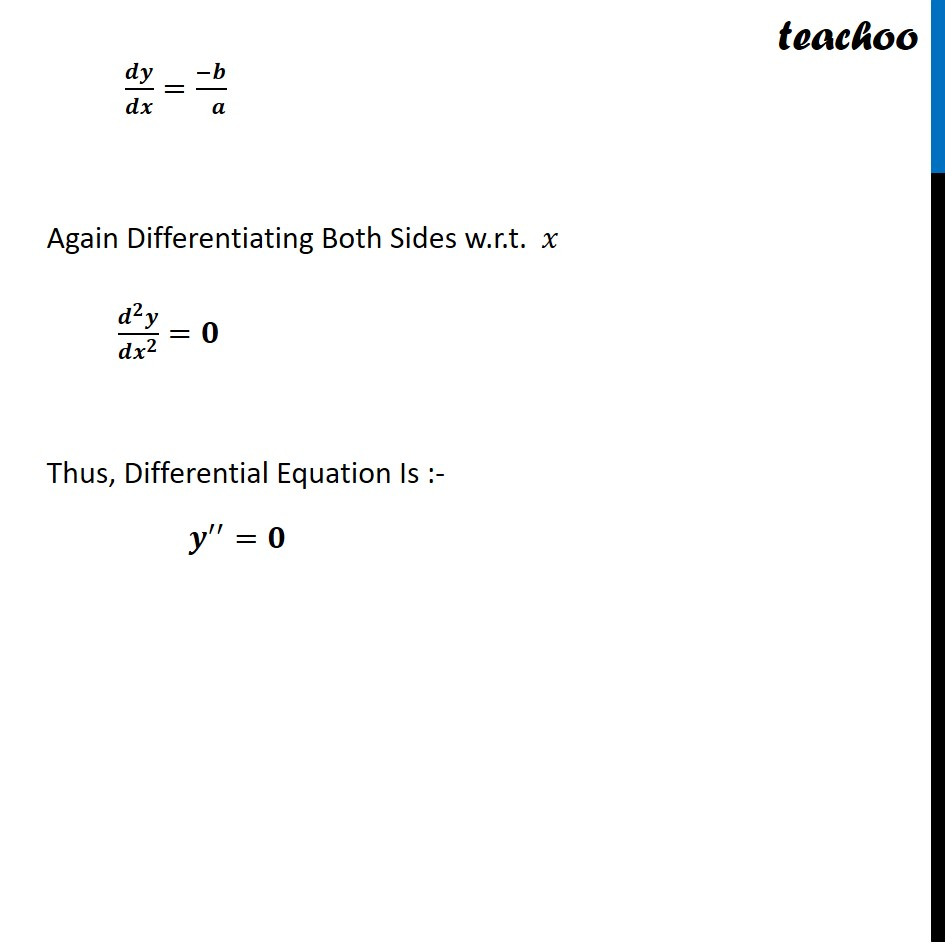Ex 9.3

Chapter 9 Class 12 Differential Equations (Term 2)
Serial order wise### Transcript

Ex 9.3, 1 Form a differential equation representing the given family of curves by eliminating arbitrary constants 𝑎 and 𝑏. 𝑥/𝑎+𝑦/𝑏=1 Here , we eliminate constants by Differentiating both sides w.r.t.x Now, 𝑥/𝑎+𝑦/𝑏=1 Differentiating Both Sides w.r.t. 𝑥 1/𝑎+1/𝑏.𝑑𝑦/𝑑𝑥=0 1/𝑏.𝑑𝑦/𝑑𝑥=(−1)/( 𝑎) The number of times we differentiate is equal to number of constants 𝒅𝒚/𝒅𝒙=(−𝒃)/( 𝒂) Again Differentiating Both Sides w.r.t. 𝑥 (𝒅^𝟐 𝒚)/(𝒅𝒙^𝟐 )=𝟎 Thus, Differential Equation Is :- 𝒚^′′=𝟎